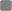###Author Topic: Autocorrelation versus cross-correlation  (Read 2101 times)

####Manda

• New Linguist
•• Posts: 1##### Autocorrelation versus cross-correlation
« on: March 02, 2015, 06:43:06 PM »
Hello,

I am analyzing segments of speech that are between 9 and 45 seconds long. I am obtaining pitch (min, max, mean, median, SD) and jitter (local) from the voice report. When I obtain the voice report it gives me a warning and states that the measurements may be imprecise and I should switch my analysis method from autocorrelation to cross-correlation. I am not sure why this is the case. Would you recommend I switch to cross-correlation or keep it on autocorrelation? Why is one method better than the other? Are my speech segments an appropriate length?

Thank you,
Amanda

####jkpate##### Re: Autocorrelation versus cross-correlation
« Reply #1 on: March 04, 2015, 07:28:27 AM »
Cross-correlation can be more robust than auto-correlation when analyzing short stretches of speech. Regardless of the total duration of your segment of speech, a voice report requires analyzing short sub-sequences. This is because jitter involves the difference between consecutive periods, and if you compute f0 over long stretches, you've already averaged out the differences between consecutive periods.

The service this board uses to render math appears to be having some kind of technical problem. I'll explain why cross-correlation can be more robust than auto-correlation more clearly once the service goes back up. But here's an attempt to explain without mathematical notation. Remember that a cross-correlation has three arguments: two signals f and g, and a lag tau. The cross-correlation of the two signals at a particular lag is an infinite sum over all t of the product of f(t) and g(t-tau): \sum_t^\infty f(t)g(t-tau) . We estimate f0 in the autocorrelation approach by setting f and g to be the same signal, call it h. The largest autocorrelation value occurs when tau is zero, because h(t) = h(t-0) for all t. The second largest autocorrelation value should usually be the wavelength of the fundamental frequency, but things like random noise can interfere.

In practice, we do not have an infinite sum, because we can only gather so many air pressure measurements before the signals change. To deal with this, practical applications will 'window' each signal. At the midpoint of the window, the signal is multiplied by 1, and it gets multiplied by smaller numbers as you get further away from (before and after) the midpoint. Outside of the window, the scaled measurements are 0.

However, this windowing can cause a problem for the autocorrelation approach. When tau gets to be close to the width of the window, either h(t) or h(t-tau) will be scaled towards zero or actually fall outside of the window, and the sum of each h(t-tau) will be artificially small. This means that the windowed autocorrelation will give shorter peaks that are more likely to be lost in noise when tau approaches the window size. This problem can become especially prevalent when the window is very narrow, as is the case when analyzing short stretches of speech to estimate variation in adjacent periods.

The cross-correlation approach to f0 estimation addresses this by essentially windowing the lagged signals separately. f is set to be the speech signal windowed around the time period of interest, and g is set to be the speech signal windowed about the lag point. This cross-correlation approach essentially decouples the choice of window size from the choice of the range of pitch periods to consider, and allows the algorithm to use the same number of non-scaled samples for both short and long lags.

All models are wrong, but some are useful - George E P Box